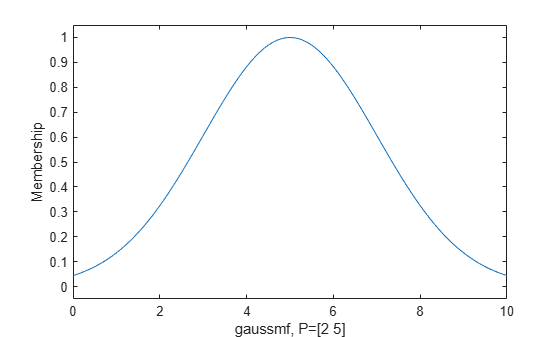# gaussmf

Gaussian membership function

## Syntax

``y = gaussmf(x,params)``

## Description

This function computes fuzzy membership values using a Gaussian membership function. You can also compute this membership function using a `fismf` object. For more information, see fismf Object.

A Gaussian membership function is not the same as a Gaussian probability distribution. For example, a Gaussian membership function always has a maximum value of `1`. For more information on Gaussian probability distributions, see Normal Distribution (Statistics and Machine Learning Toolbox).

example

````y = gaussmf(x,params)` returns fuzzy membership values computed using the following Gaussian membership function:$f\left(x;\sigma ,c\right)={e}^{\frac{-{\left(x-c\right)}^{2}}{2{\sigma }^{2}}}$To specify the standard deviation, σ, and mean, c, for the Gaussian function, use `params`.Membership values are computed for each input value in `x`.```

## Examples

collapse all

Specify input values across the universe of discourse.

`x = 0:0.1:10;`

Evaluate membership function for the input values.

`y = gaussmf(x,[2 5]);`

Plot the membership function.

```plot(x,y) xlabel('gaussmf, P=[2 5]') ylabel('Membership') ylim([-0.05 1.05])```## Input Arguments

collapse all

Input values for which to compute membership values, specified as a scalar or vector.

Membership function parameters, specified as the vector [σ c], where σ is the standard deviation and c is the mean.

## Output Arguments

collapse all

Membership value returned as a scalar or a vector. The dimensions of `y` match the dimensions of `x`. Each element of `y` is the membership value computed for the corresponding element of `x`.

## Alternative Functionality

### `fismf` Object

You can create and evaluate a `fismf` object that implements the `gaussmf` membership function.

```mf = fismf("gaussmf",P); Y = evalmf(mf,X);```

Here, `X`, `P`, and `Y` correspond to the `x`, `params`, and `y` arguments of `gaussmf`, respectively.

## Version History

Introduced before R2006a

expand all